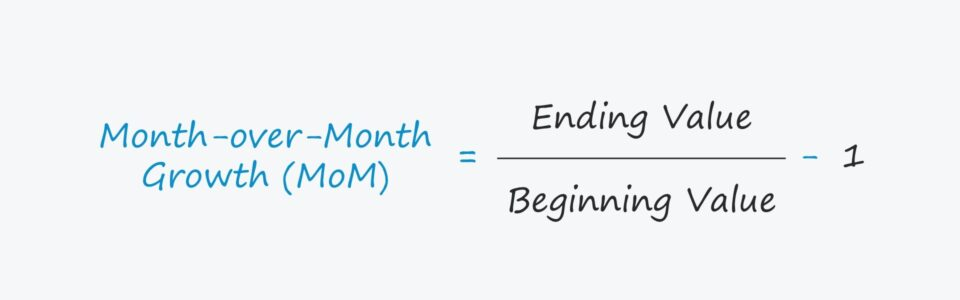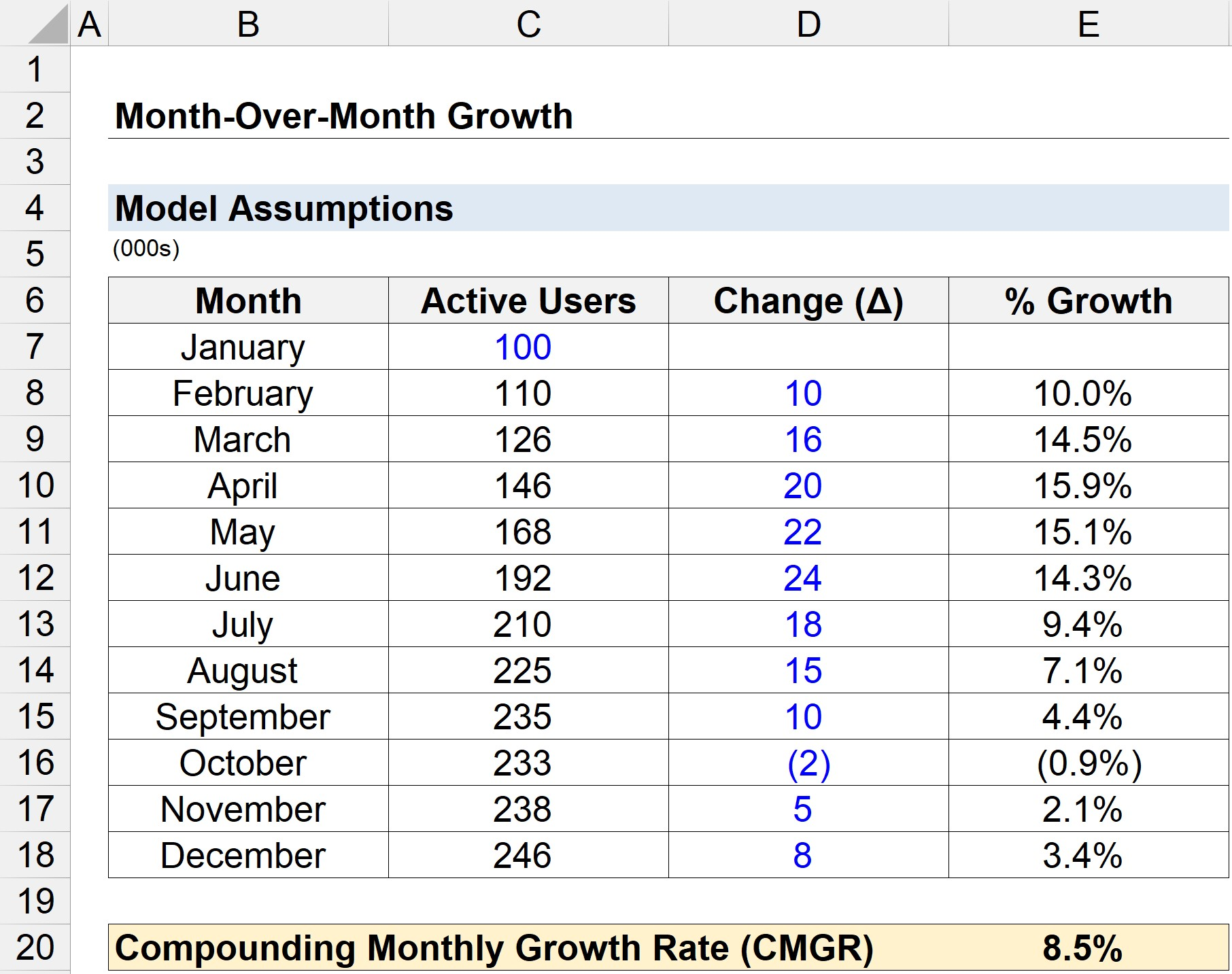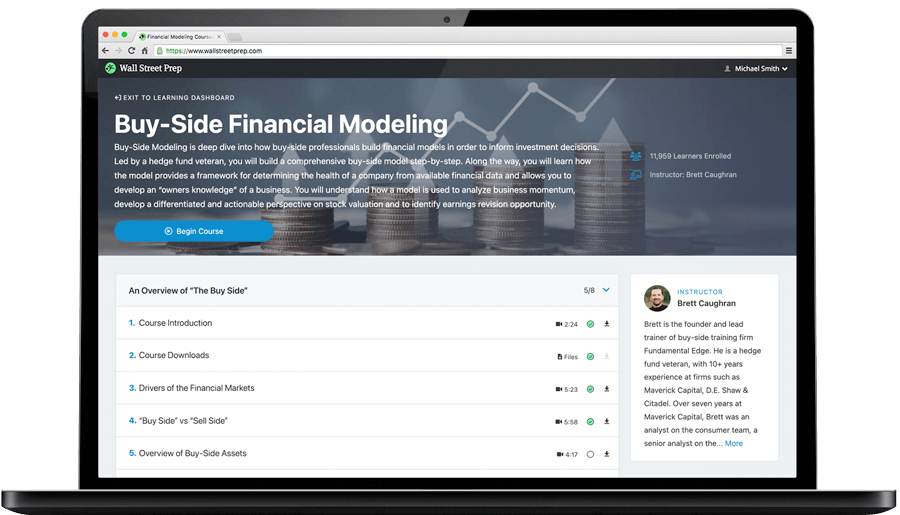Welcome to Wall Street Prep! Use code at checkout for 15% off.# Month over Month Growth (M/M)

Guide to Understanding Month over Month Growth (M/M)## How to Calculate Month over Month Growth (Step-by-Step)

The month over month growth rate shows the change in the value of a metric – such as revenue or the number of active users – expressed as a percentage of the prior month’s value.

For mature companies, one of the main use-cases for calculating the monthly growth rate is to understand the cyclicality of a company’s performance.

The monthly growth rate is also important to track for early-stage companies since metrics such as the run rate revenue are based on recent performance due to the high growth rates of such companies.

Calculating the month-over-month growth rate is a two-step process:

1. The first step is to divide the current month’s value by the prior month’s value
2. In the second step, one is subtracted from the result from the previous step.

## Month over Month Growth Formula (M/M)

The monthly growth rate formula is as follows.

Month over Month Growth = (Current Month Value / Prior Month Value) – 1

The result will be in the form of a fraction, so the resulting value must then be multiplied by 100 to express the metric as a percentage (%).

Another method to calculate the monthly growth rate is to subtract the prior month’s value from the current month’s value and then divide it by the prior month’s value.

Month over Month Growth = (Current Month Value – Prior Month Value) / Prior Month Value

For instance, let’s consider if a company had 200 active users in January and 240 in February.

Using the equation below, we can calculate that the monthly growth rate in active users was 20%.

• Monthly Growth Rate = (240 / 200) – 1 = 0.20, or 20%

## Compounding Monthly Growth Rate Formula (CMGR)

The compounding monthly growth rate (CMGR) refers to the average month-over-month growth of a metric.

The CMGR formula is shown below.

CMGR = (Final Month Value / Initial Month Value) ^ (1 / # of Months) – 1

For example, let’s say a mobile application company is attempting to calculate the CMGR of its monthly active users (MAUs).

At the end of January 2022, there was a total of 10,000 users, which grew to 20,000 active users by the end of December 2022.

If we enter those assumptions into the formula, we calculate 6.5% as the CMGR. The interpretation is that on average, between January and December 2022, users grew by 6.5% per month.

• CMGR = 20,000 / 10,000 ^(1/11) – 1
• CMGR = 6.5%

## Month over Month Growth Calculator

We’ll now move to a modeling exercise, which you can access by filling out the form below.Submitting...

## Month over Month Growth Calculation Example

Suppose you’re tasked with calculating the monthly growth rate of a company’s active user base.

In January, the company had a total of 100k active users, with the net additions (and losses) in all the subsequent months summarized below.

• February: +10k
• March: +16k
• April: +20k
• May: +22k
• June: +24k
• July: +18k
• August: +15k
• September: +10k
• October: –2k
• November: +5k
• December: +8k

Starting from January, if we add the monthly change for each month, we arrive at the following active user counts.

Month Active Users % M/M Growth
January 100k n.a. M/M
February 110k 10.0% M/M
March 126k 14.5% M/M
April 146k 15.9% M/M
May 168k 15.1% M/M
June 192k 14.3% M/M
July 210k 9.4% M/M
August 225k 7.1% M/M
September 235k 4.4% M/M
October 233k (0.9%) M/M
November 238k 2.1% M/M
December 246k 3.4% M/M

Moreover, we can divide the current month by the prior month and then subtract one to arrive at the month-over-month growth rates, as shown above in the far right column.

We can conclude that the company experienced the strongest growth around the Spring during March to June, with growth beginning to decline in the Fall.

Next, the compounding monthly growth rate (CMGR) can be calculated using the equation shown below.

• Compounding Monthly Growth Rate (CMGR) = (246k / 100k)^(1/11) – 1
• CMGR = 8.5%

On average, the Company’s user count grew by 8.5% per month between January and December.Step-by-Step Online Course

### Learn Buy-Side (Hedge Fund) Modeling

Led by a former hedge fund PM (Maverick, Citadel, DE Shaw, Schonfeld), this program begins where financial modeling training ends — with a deep-dive into how buy-side analysts build financial models to make key investment decisions.